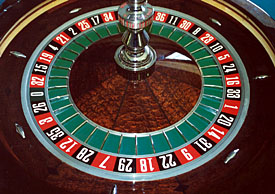Categories

## Why Casinos Don’t Lose MoneySo, how does this tie into casinos?  Let’s take the roulette wheel as our example.  There are 37 total numbers.  18 reds, 18 blacks, and 1 green.  If you guess red or black correctly, you’ll get a 1:1 payout (ie: If you bet $1, you’ll get back$2, thereby winning $1). If the wheel lands on green (0), both red and black lose. This is where the casino gets it’s edge in this particular gamble. Let’s calculate the expected value of a$1 bet on red.

$$E[X] = \frac{18}{37}(\1)+\frac{18}{37}(-\1)+\frac{1}{37}(\-1) = -\.03$$

What this means is you have an 18/37 chance of winning $1 (if it lands on red), and 18/37 chance of losing$1 (if it lands on black), and a 1/37 chance of losing $1 (if it lands on green) The expected profit for playing this game is negative 2 cents. Now, sometimes you’ll win, and sometimes you’ll lose, but if you play enough times, you’ll be averaging a loss of 23 cents per round. This is where the law of large numbers comes into play. As long as enough people are playing, the house will be averaging a profit of 2 cents for every dollar bet on that roulette table. Question: What is the expected value of correctly guessing a specific number? There are 37 numbers, but the payout is 35:1 (You get paid$35 for each dollar you bet)  Based on this answer, is it smarter to try guessing the color or guessing the number?

Categories

## Quitting While You’re Ahead? Random Walks & Markov ChainsQuestion:  What if you walked up to a “fair” casino game (50/50 odds of winning) that pays even odds with $10,000, and said you would quit as soon as you’re up$1,000 ? (ie: You either walk out with $11,000 or keep playing until you lose everything) What are the odds of you leaving the casino with a$1000 profit?

Markov Chain can virtually simulate many random walks of this experiment.  With a large enough sample size, you can get an accurate sense of the odds of walking out with $1,000.The following code runs this simulation as many times as you want, and tells you how many times you got to$11,000.

#!/usr/local/bin/perl
#!/opt/bin/perl

use Getopt::Long;
use strict;

GetOptions("f:s", "debug", "v");

my $current_round = 0; my$starting_amt = 10;
my $lost = 0; my$won = 0;
my $current_amt; my$x;
my $low_bound = 0; my$high_bound = 11;

while ($current_round < 100) { while ($current_amt > $low_bound &&$current_amt < $high_bound) { #generate a 1 or 0$x = int(rand(1)+.5);

#adjust to either +1 or -1
if ($x == 0) {$x = -1;
}

# update total
$current_amt -=$x;

# print current total
print "$current_amt "; } print "\n"; #increment rounds won or lost... if ($current_amt == 0) {
$lost++; } else {$won++;
}

#reset current amount for next round
$current_amt =$starting_amt;

$current_round++; } print "\nWon =$won\n";
print "Lost = $lost\n"; Answer: Doing 100 rounds is a large enough sample size to get an accurate result. On average, you’ll walk out with$11,000 about 90% of the time you try this experiment.  Why is this still a bad idea?  In practice, gamblers rarely quit while they are ahead, and you still have that 10% odds of a total washout.  Recall, the expected value of this game is still break even.

Here is a sample output of 10 rounds:

Won = 9
Lost = 1